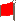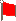NextProgram Repair Shop184

# get_computer_choice

```   195     sub get_computer_choice {
196        my @available_choices = @_;
197        my \$length = @available_choices;
198        my \$number = int(rand() * (\$length - 1));
199        my \$choice = \$available_choices[\$number];
200        return \$choice;  ## this will be a coordinate, in the form of \$x\$y from \$z above
201     }
```
• This is wasteful

```           sub get_computer_choice {
my \$length = @_;
my \$number = int(rand() * (\$length - 1));
my \$choice = \$_[\$number];
return \$choice;  ## this will be a coordinate, in the form of \$x\$y from \$z above
}
```
```           sub get_computer_choice {
my \$number = int(rand() * (@_ - 1));
return \$_[\$number];
}
```

 NextCopyright © 2001 M. J. Dominus